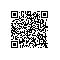# 五大经典算法之回溯法

### 一、基本概念

回溯法，又称为试探法，按选优条件向前不断搜索，以达到目标。但是当探索到某一步时，如果发现原先选择并不优或达不到目标，就会退回一步重新选择，这种达不到目的就退回再走的算法称为回溯法。

### 二、基本思想

对于可以使用回溯法来解决的问题，首先可以将其解空间可以看成一棵解空间树。在回溯法中，每次扩大当前部分解时，都面临一个可选的状态集合（所有的子树），每个树结点代表一个可能的部分解。
回溯法对任一解的生成，一般都采用逐步扩大解的方式。每前进一步，都试图在当前部分解的基础上扩大该部分解。它在问题的状态空间树中，从开始结点（根结点）出发，以深度优先搜索整个状态空间。这个开始结点成为活结点，同时也成为当前的扩展结点。在当前扩展结点处，搜索向纵深方向移至一个新结点。这个新结点成为新的活结点，并成为当前扩展结点。如果在当前扩展结点处不能再向纵深方向移动，则当前扩展结点就成为死结点。此时，应往回移动（回溯）至最近的活结点处，并使这个活结点成为当前扩展结点。回溯法以这种工作方式递归地在状态空间中搜索，直到找到所要求的解或解空间中已无活结点时为止。

### 三、解题步骤(思路)

1. 针对给定的问题，定义问题的解空间；
2. 确定易于搜索的解空间结构；
3. 以深度优先方式搜索解空间，并且在搜索过程中用剪枝函数避免无效搜索。（这里的剪枝函数就是判断该结点是否满足问题题设，如果满足则向下搜索，不满足则在此剪枝

### 四、算法框架

1. 递归实现：
变量解释：
x：存储试探解的数组
n：解空间树的层数
i：搜索目前所达到的层数
start：子节点解空间的最小值
end：子节点解空间的最大值

int x[n];
void backtrack (int i) {
if (i > n) {
回溯结束；
} else {
// 这里回溯子节点的解空间为start~end
for (j = start; j <= end; j++) {
// 满足条件，向下搜索
if (j满足题设条件) {
x[i] = j;
backtrack(i+1);
// 不满足条件，在此剪枝（即回溯）
} else {
}
}
}
}


2. 非递归实现：
变量解释：
x：存储试探解的数组
n：解空间树的层数
i：搜索目前所达到的层数
start：子节点解空间的最小值
end：子节点解空间的最大值

void f_backtrack(int i) {
//初始化解向量
for (int j = 0; j < n; j++) {
x[j] = 1;
}
while (i >= 1) {
while (x[i] <= n) {
if (place(i)) {
if (i == n) {
回溯结束；
break;
// 满足条件，向下搜索
} else {
i++;
x[i] = 1;
}
// 不满足条件，在此剪枝（即回溯）
} else {
x[i]++;
}
}
//遍历完子节点解空间后，向上剪枝（即回溯）
x[i] = 1;
i--;
x[i]++;
}
}


### 五、经典实现

八皇后问题，是一个古老而著名的问题，是回溯算法的典型例题。该问题是十九世纪著名的数学家高斯1850年提出：
在8X8格的国际象棋上摆放八个皇后，使其不能互相攻击，即任意两个皇后都不能处于同一行、同一列或同一斜线上（斜率为1），问有多少种摆法。高斯认为有76种方案。1854年在柏林的象棋杂志上不同的作者发表了40种不同的解，后来有人用图论的方法解出92种结果。

#include <iostream>
using namespace std;
int n;
int *x;
int sum;
bool place(int k)
{
for (int j = 1; j < k; j++)
if (abs(x[k] - x[j]) == abs(k - j) || x[j] == x[k])
return false;
return true;
}

void output()
{
sum++; //sum为所有的可行的解
for (int m = 1; m <= n; m++)
{
cout << "<" << m << "," << x[m] << ">"; //这一行用输出当递归到叶节点的时候，一个可行解
}
cout << endl;
}

void f_backtrack(int i)
{
for (int j = 0; j < n; j++)
{ //初始化解向量
x[j] = 1;
}
while (i >= 1)
{
while (x[i] <= n)
{
if (place(i))
{ //得到可行解
if (i == n)
{
output();
break;
} //得到最终可行解，退出
else
{ //得到部分可行解，搜索下一行
i++;
x[i] = 1;
}
}
else
{ //当前解不可行
x[i]++;
}
}
x[i] = 1;
i--;
x[i]++; //回溯
}
}

void backtrack(int i)
{
if (i > n)
{
output();
}
else
{
for (int j = 1; j <= n; j++)
{
x[i] = j;
if (place(i))
{
backtrack(i + 1);
}
else
{
}
}
}
}

int main()
{
n = 8;
sum = 0;
x = new int[n + 1];
for (int i = 0; i <= n; i++)
x[i] = 0;
backtrack(1);
cout << "方案共有" << sum << endl;
}使用钉钉扫一扫加入圈子
+ 订阅